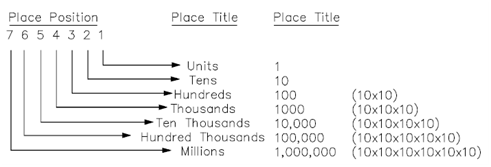## Decimal Numbering System Assignment Help

Assignment Help: >> Basic Arithmetic Operations - Decimal Numbering System

Decimal Numbering System:

The decimal numbering system uses ten symbols known as digits, every digit representing a number. Those given symbols are 0, 1, 2, 3, 4, 5, 6, 7, 8 and 9.  The symbols are called as the numbers zero, one, two, three, etc.  Through using combinations of 10 symbols, an infinite amount of numbers could be created.  For example, we could group 5 and 7 together for the number 57 or 2 and 3 together for the number 23.  Place values of the digits are multiples of ten and provided place titles as below:Numbers in the decimal system might be categorized as integers or fractions.  An integer is a whole number like as 1, 2, 3, . . . 10, 11, . . .   A fraction is a element of a whole number, & that is expressed as a ratio of integers, like as 1/2, 1/4, or 2/3.

An even integer is an integer that could be exactly divided via 2, like as 4, 16, and 30.  All other integers are known as odd, such as 3, 7, and 15.  A number can be determined to be either odd/even through noting the digit in the units place position.  If this digit is even after that the number is even; if it is odd, then the number is odd.  Numbers that end in 0, 2, 4, 6, 8 are even, and numbers ending in 1, 3, 5, 7, 9 are odd.  Zero (0) is even.